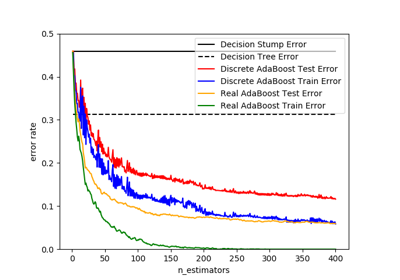# sklearn.metrics.zero_one_loss¶

sklearn.metrics.zero_one_loss(y_true, y_pred, normalize=True, sample_weight=None)[source]

Zero-one classification loss.

If normalize is True, return the fraction of misclassifications (float), else it returns the number of misclassifications (int). The best performance is 0.

Read more in the User Guide.

Parameters: y_true : 1d array-like, or label indicator array / sparse matrix Ground truth (correct) labels. y_pred : 1d array-like, or label indicator array / sparse matrix Predicted labels, as returned by a classifier. normalize : bool, optional (default=True) If False, return the number of misclassifications. Otherwise, return the fraction of misclassifications. sample_weight : array-like of shape = [n_samples], optional Sample weights. loss : float or int, If normalize == True, return the fraction of misclassifications (float), else it returns the number of misclassifications (int).

Notes

In multilabel classification, the zero_one_loss function corresponds to the subset zero-one loss: for each sample, the entire set of labels must be correctly predicted, otherwise the loss for that sample is equal to one.

Examples

>>> from sklearn.metrics import zero_one_loss
>>> y_pred = [1, 2, 3, 4]
>>> y_true = [2, 2, 3, 4]
>>> zero_one_loss(y_true, y_pred)
0.25
>>> zero_one_loss(y_true, y_pred, normalize=False)
1


In the multilabel case with binary label indicators:

>>> zero_one_loss(np.array([[0, 1], [1, 1]]), np.ones((2, 2)))
0.5


## Examples using sklearn.metrics.zero_one_loss¶Discrete versus Real AdaBoost